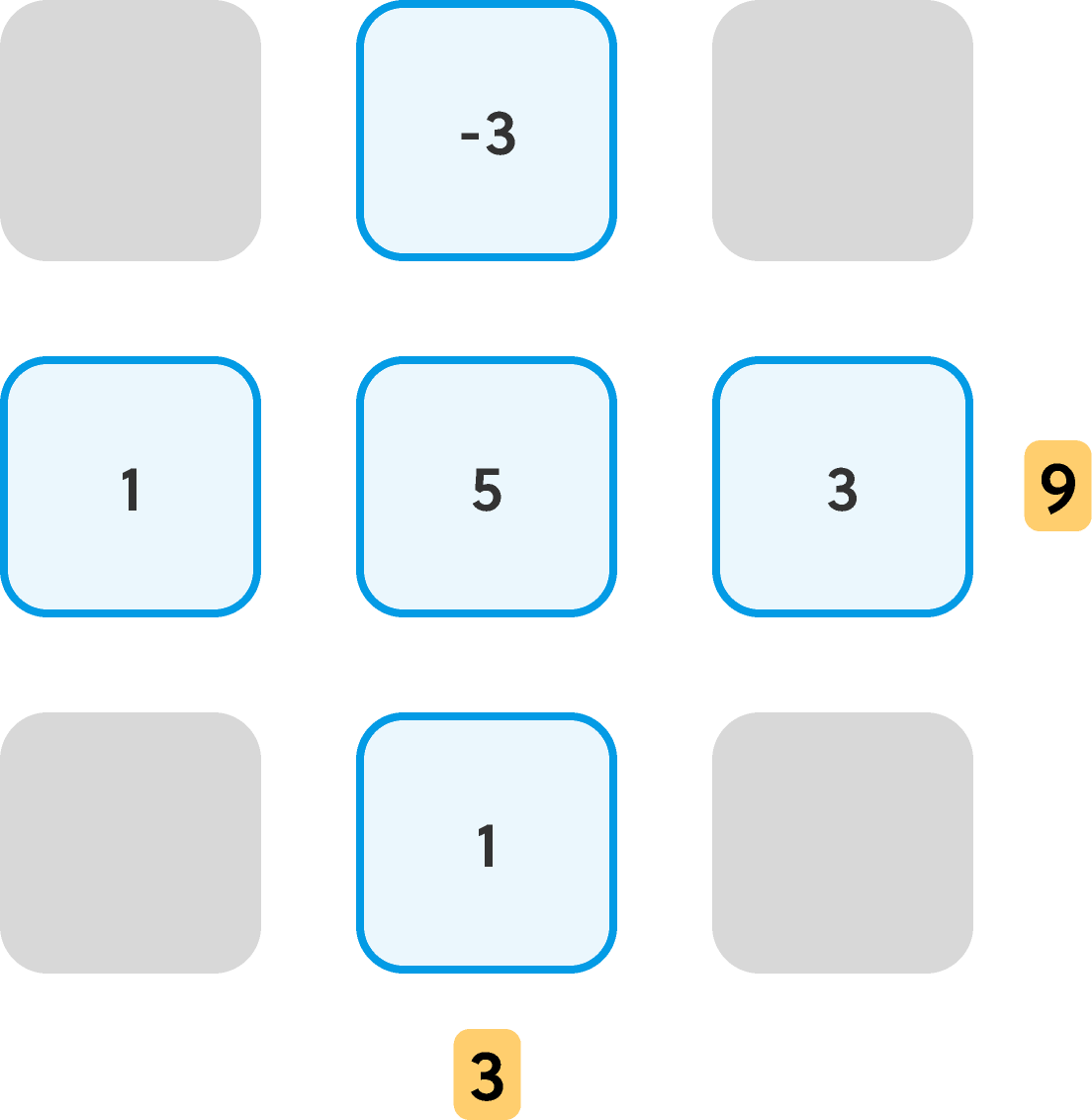Back

## The Nine Nine Plus

Sometimes, the best way to solve a problem is to start by just doing something with it. Then slow down, and think about what you're doing. For example, think about what you needed to do to solve just one part of the problem.

Here's a warm-up challenge. Try it yourself. Drag the numbered tiles up into the plus.

Note that the sum of all of the available tiles is $0$, and yet the goal is to make the row sum and the column sum both positive. When they first see this puzzle, some people refuse to even try to solve it. They glance at it and claim that it's impossible... we promise, it's not.

If you start by just filling in the row so that it sums to $1,$ how many different ways are there to do that?

After you've gotten a three-tile row to equal $1,$ try rearranging that row so that you can also make the three-tile column equal $1$ using the center tile of the row and the two tiles you didn't use for the row.

Here's our solution:

Your solution might look a bit different, but as long as the $0$ and $-1$ are together in the same row or column and the $1$ and $-2$ are together in the other, both sums will be correct. There are actually eight slightly different-looking solutions.

So we've shown that it's definitely possible to arrange $-2, -1, 0, 1,$ and $2$ into a plus that has a positive row and column sum. Let's take that victory one step further: is it true that, if you arrange any five number tiles in a plus and put a positive value in the center, the row sum plus the column sum will be larger than the sum of the five tiles? Can you prove why this is true? Here's another example:And, to wrap things up, here's a bonus challenge that's a fair bit more complex than the plus-arrangement. However, it can be solved using the same algebraic strategies that make the puzzle above and today's challenge solvable. Good luck!

# Today's Challenge

Is it possible to arrange five square tiles numbered $1, 2, 3, 4,$ and $5$ into a "plus" so that the sum of the three-tile column and the sum of the three-tile row are both equal to $9?$

Try it yourself by dragging the five numbered tiles up into the plus.×